Subsections

# Phase Transitions of Ising Model

## Zero Field. Curie Point

Let H=0 (symmetric case). Entropy wants spins to be disoriented, energy--to be parallel

• At large T entropy wins: M=0
• At small T energy wins: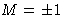• In the Curie point Tc--phase transition. This is a second order phase transition (why?).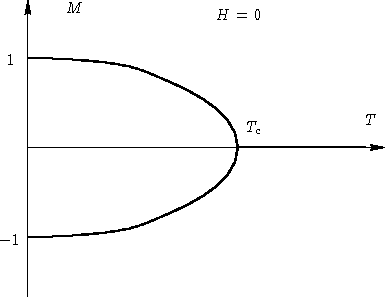## Non-Zero Field. Susceptibility

If, magnetization M is parallel to H. There is a function M(H,T). What happens at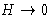?

• If T>Tc, the system is disordered at H=0: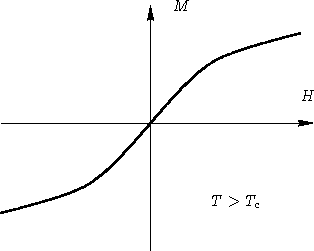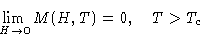• If T<Tc, the system is ordered at H=0: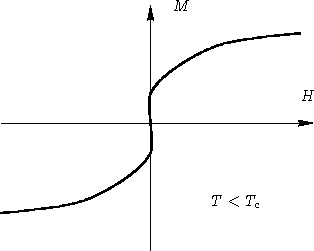• If T=Tc, the points M0 and -M0 coincide--the tangent is vertical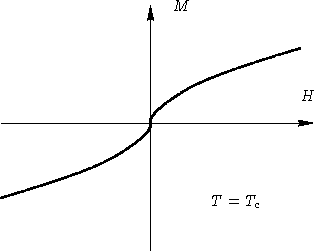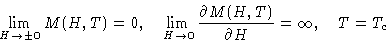In critical point susceptibility is infinity!

Since amplitude of fluctuations is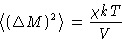the fluctuations infinitely grow at Tc!

## Phase Diagram© 1997 Boris Veytsman and Michael Kotelyanskii
Mon Oct 13 22:07:20 EDT 1997